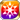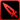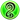# On Passion We SoarTrue fire, faux fire; true justice, and false justice. I believed that charging with reckless abandon was inefficient, but what is truly important is that one does not waver. "We might have different notions about justice, but that will not stop me. I will not hesitate, but simply carry your ideas with me!" I cannot allow the tragedy that befell Wadatsumi to be repeated. Upon this amulet, I swear this oath both unto the Captain, and unto you. For that is the nature of the justice we share. (Seida's Unsent Letter to Monzein)

### On Passion We Soar

Raises HP by 30%/PATK by 30%/DEX by 30%/AGI by 5%/Strong vs Greed by 30%/Strong vs Gluttony by 30% for the Memento group "Holy Guard"

Target Buff (SelfSide) (CardLsSkill)
Stats
TypeMinMax
HP+30%+30%
PATK+30%+30%
DEX+30%+30%
AGI+5%+5%
Strong vs Greed+30+30
Strong vs Gluttony+30+30

## Stats

#### Simple stats

TypeLvl 1Lvl 30Lvl 40
PATK+8+20+25

LevelPATK
1+8
2+8
3+8
4+9
5+9
6+10
7+10
8+11
9+11
10+11
LevelPATK
11+12
12+12
13+13
14+13
15+14
16+14
17+14
18+15
19+15
20+16
LevelPATK
21+16
22+17
23+17
24+18
25+18
26+18
27+19
28+19
29+20
30+20
LevelPATK
31+21
32+21
33+21
34+22
35+22
36+23
37+23
38+24
39+24
40+25

## Group skills

### The Miracle of Saga

#### Simple stats

TypeLvl 1Lvl 30Lvl 40
PATK+10+32+40
Initial Jewels+2+6+8

#### Detailed stats

LevelPATKInitial Jewels
1+10+2
2+10+2
3+11+2
4+12+2
5+13+2
6+13+2
7+14+2
8+15+3
9+16+3
10+16+3
LevelPATKInitial Jewels
11+17+3
12+18+3
13+19+3
14+20+4
15+20+4
16+21+4
17+22+4
18+23+4
19+23+4
20+24+4
LevelPATKInitial Jewels
21+25+5
22+26+5
23+26+5
24+27+5
25+28+5
26+29+5
27+30+6
28+30+6
29+31+6
30+32+6
LevelPATKInitial Jewels
31+33+6
32+33+6
33+34+6
34+35+7
35+36+7
36+36+7
37+37+7
38+38+7
39+39+7
40+40+8

#### Limit Break

TypeLB1LB2LB3LB4LB5
PATK+6+10+15+19+24
Initial Jewels+1+2+3+4+5

### The Miracle of Saga

#### Simple stats

TypeLvl 1Lvl 30Lvl 40
PATK+10+32+40
Initial Jewels+2+6+8

#### Detailed stats

LevelPATKInitial Jewels
1+10+2
2+10+2
3+11+2
4+12+2
5+13+2
6+13+2
7+14+2
8+15+3
9+16+3
10+16+3
LevelPATKInitial Jewels
11+17+3
12+18+3
13+19+3
14+20+4
15+20+4
16+21+4
17+22+4
18+23+4
19+23+4
20+24+4
LevelPATKInitial Jewels
21+25+5
22+26+5
23+26+5
24+27+5
25+28+5
26+29+5
27+30+6
28+30+6
29+31+6
30+32+6
LevelPATKInitial Jewels
31+33+6
32+33+6
33+34+6
34+35+7
35+36+7
36+36+7
37+37+7
38+38+7
39+39+7
40+40+8

#### Limit Break

TypeLB1LB2LB3LB4LB5
PATK+6+10+15+19+24
Initial Jewels+1+2+3+4+5

### Holy Guard's Lightning Flash

#### Simple stats

TypeLvl 1Lvl 30Lvl 40
AGI+2+4+6
Thunder Res+2+6+8

#### Detailed stats

LevelAGIThunder Res
1+2+2
2+2+2
3+2+2
4+2+2
5+2+2
6+2+2
7+2+2
8+2+3
9+2+3
10+2+3
LevelAGIThunder Res
11+3+3
12+3+3
13+3+3
14+3+4
15+3+4
16+3+4
17+3+4
18+3+4
19+3+4
20+3+4
LevelAGIThunder Res
21+4+5
22+4+5
23+4+5
24+4+5
25+4+5
26+4+5
27+4+6
28+4+6
29+4+6
30+4+6
LevelAGIThunder Res
31+5+6
32+5+6
33+5+6
34+5+7
35+5+7
36+5+7
37+5+7
38+5+7
39+5+7
40+6+8

#### Limit Break

TypeLB1LB2LB3LB4LB5
AGI+1+1+2+3+4
Thunder Res+2+2+3+4+5

### Efficient Heresy

#### Simple stats

TypeLvl 1Lvl 30Lvl 40
Strong vs Templar+7+16+20

#### Detailed stats

LevelStrong vs Templar
1+7
2+7
3+7
4+8
5+8
6+8
7+9
8+9
9+9
10+10
LevelStrong vs Templar
11+10
12+10
13+11
14+11
15+11
16+12
17+12
18+12
19+13
20+13
LevelStrong vs Templar
21+13
22+14
23+14
24+14
25+15
26+15
27+15
28+16
29+16
30+16
LevelStrong vs Templar
31+17
32+17
33+17
34+18
35+18
36+18
37+19
38+19
39+19
40+20

#### Limit Break

TypeLB1LB2LB3LB4LB5
Strong vs Templar+4+6+8+10+12

#### Max Limit Break

TypeLB5
Jewels Spent Rate-16

### Pride of the Alize People

#### Simple stats

TypeLvl 1Lvl 30Lvl 40
DEX+15+41+50
Hit Rate+3+8+10
Range+1+1+1

#### Detailed stats

LevelDEXHit RateRange
1+15+3+1
2+15+3+1
3+16+3+1
4+17+3+1
5+18+3+1
6+19+3+1
7+20+4+1
8+21+4+1
9+22+4+1
10+23+4+1
LevelDEXHit RateRange
11+23+4+1
12+24+4+1
13+25+5+1
14+26+5+1
15+27+5+1
16+28+5+1
17+29+5+1
18+30+6+1
19+31+6+1
20+32+6+1
LevelDEXHit RateRange
21+32+6+1
22+33+6+1
23+34+6+1
24+35+7+1
25+36+7+1
26+37+7+1
27+38+7+1
28+39+7+1
29+40+8+1
30+41+8+1
LevelDEXHit RateRange
31+41+8+1
32+42+8+1
33+43+8+1
34+44+8+1
35+45+9+1
36+46+9+1
37+47+9+1
38+48+9+1
39+49+9+1
40+50+10+1

#### Limit Break

TypeLB1LB2LB3LB4LB5
DEX+10+15+20+25+30
Hit Rate+2+3+4+5+6

#### Max Limit Break

TypeLB5
Missile ATK Power+10

## Vision abilities

### Godwolf Fang

#### Ability base

• Slot:Action
• DMG Type:Physical
• ATK Type:Missile
• Element:Wind

Wind Missile ATK on units within area & greatly lowers Missile Res for three turns [Range: 5, Area: Square (3), Height Range: Unlimited]

Skill Effect
TypeMinMax
Attack110%131%
• Charges: 2
• Jewel Cost: 30
• Height: 99
• Select Range: Diamond
• Range: 0-5
• Select Scope: Square
• Scope: 1

#### Ability max

• Slot:Action
• DMG Type:Physical
• ATK Type:Missile
• Element:Wind

Wind Missile attack on units within area & greatly lowers Missile Res for three turns [Range: 5, Area: Square (3), Height Range: Unlimited]

Skill Effect
TypeMinMax
Attack210%210%
• Charges: 2
• Jewel Cost: 30
• Height: 99
• Select Range: Diamond
• Range: 0-5
• Select Scope: Square
• Scope: 1

### Limit Reached Bonus

#### Ability base

• Slot:Support

Revealed after Limit Reached. Raises AGI after appearing on map.

#### Ability max

• Slot:Action

Raises AGI after appearing on map.